# Laws of Electric Charges Electric Charge electric charges

• Slides: 16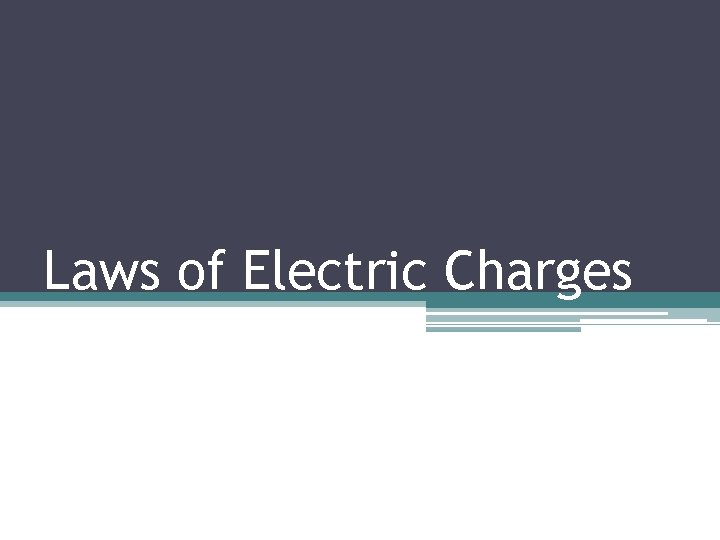Laws of Electric ChargesElectric Charge – electric charges exert forces on each other even when they are not in direct contactLaw of Electric Charges • Opposite charges attract • Similar charges repelEvery charged object creates an electric field in the space around it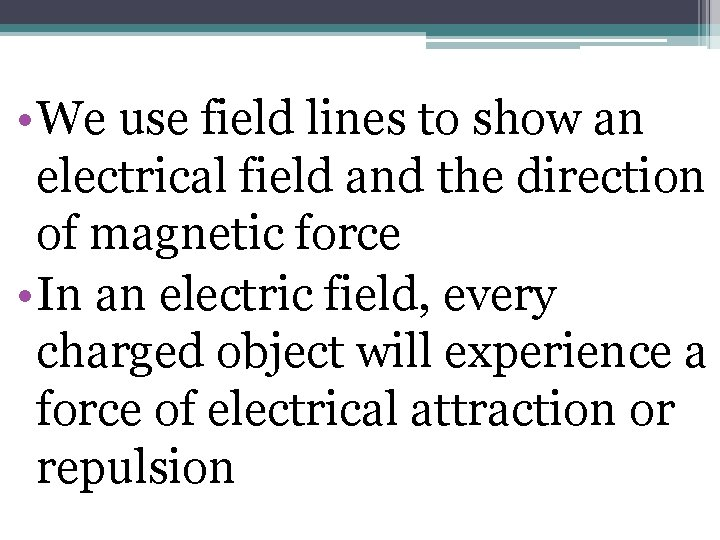• We use field lines to show an electrical field and the direction of magnetic force • In an electric field, every charged object will experience a force of electrical attraction or repulsion• The direction of a field line in the electric field is the direction of the force on a small POSITIVE charge. If negative charge, direction of the force will be the opposite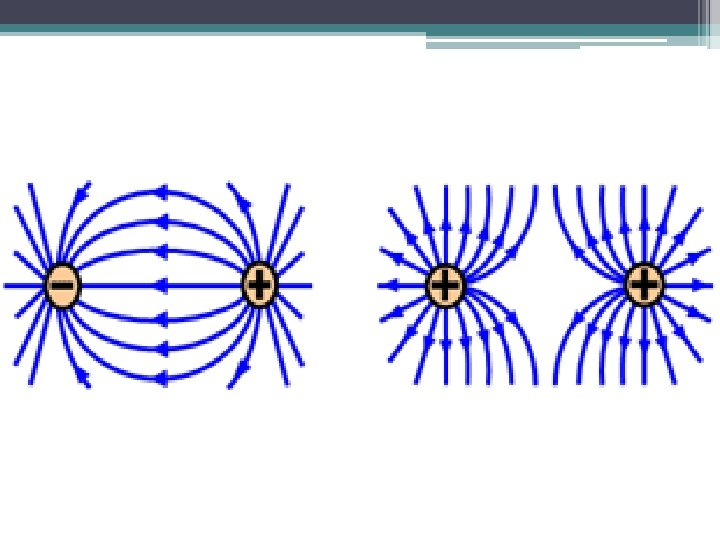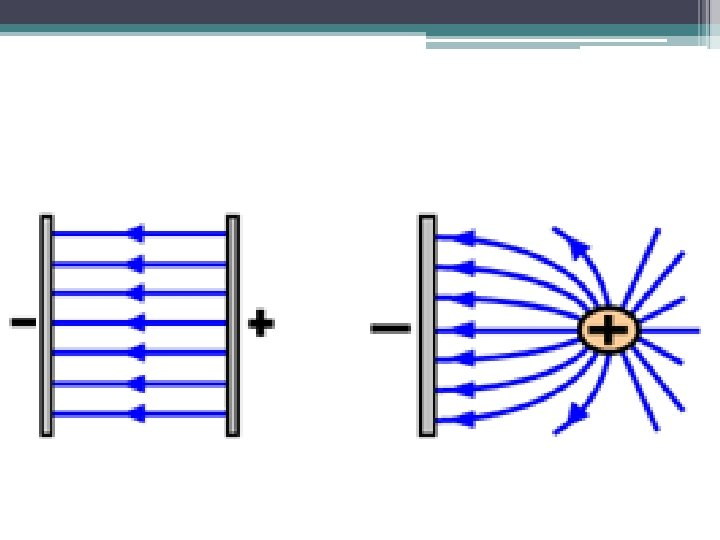Draw your own electric fields Draw the following charges in positions of your choosing (unless otherwise indicated) and create the electric field that would be present: a) Four + charges at the four points of a square b) Two + charges and a – charge at the three points of a triangle c) Three negative charges and one positive charge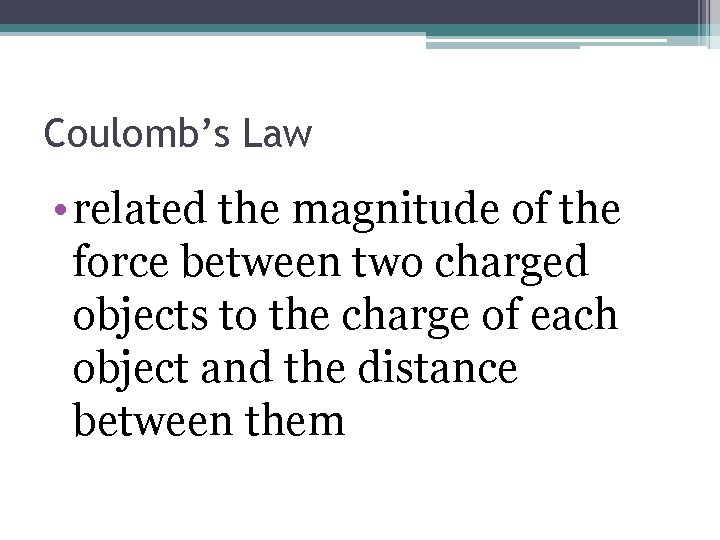Coulomb’s Law • related the magnitude of the force between two charged objects to the charge of each object and the distance between themCoulomb’s Law • Q 1, 2 – charge on each object • d – distance between the charged objects • K – constant (9. 0 x 109 Nm 2/C 2) • F – electric force between two charged objects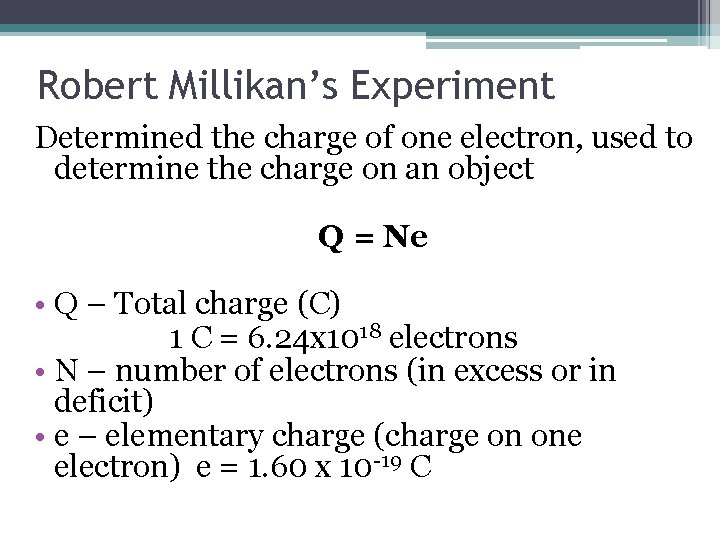Robert Millikan’s Experiment Determined the charge of one electron, used to determine the charge on an object Q = Ne • Q – Total charge (C) 1 C = 6. 24 x 1018 electrons • N – number of electrons (in excess or in deficit) • e – elementary charge (charge on one electron) e = 1. 60 x 10 -19 C• An electron has a negative elementary charge (-e) while a proton has a positive elementary charge (e)Practice Problem • What is the charge in coulombs on an object that has an excess of 6. 25 x 1019 electrons? • Ans: Q = -10 C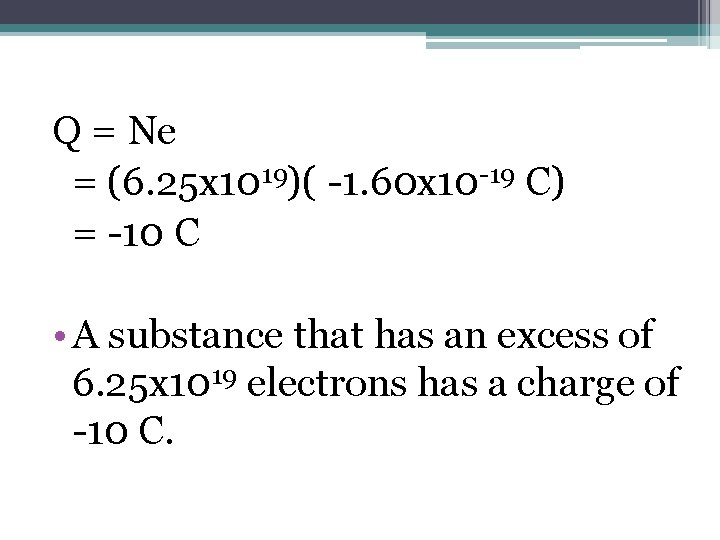Q = Ne = (6. 25 x 1019)( -1. 60 x 10 -19 C) = -10 C • A substance that has an excess of 6. 25 x 1019 electrons has a charge of -10 C.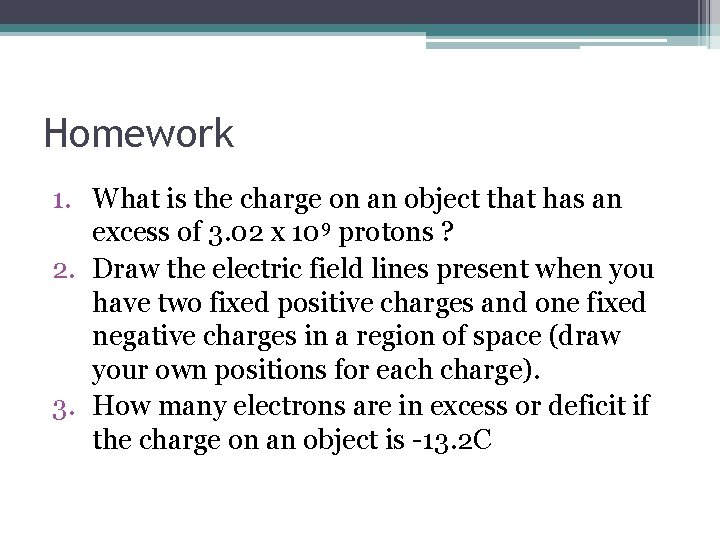Homework 1. What is the charge on an object that has an excess of 3. 02 x 109 protons ? 2. Draw the electric field lines present when you have two fixed positive charges and one fixed negative charges in a region of space (draw your own positions for each charge). 3. How many electrons are in excess or deficit if the charge on an object is -13. 2 C